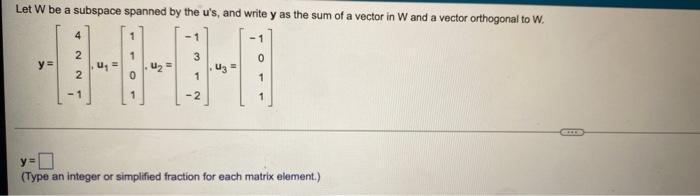Question:

# Let W be a subspace spanned by the u's, and write y as the sum of a vector in W and a vector orthogonal to W. y = 4 u₁ = 1 u₂ = -1 u₃ = -1 2 1Let W be a subspace spanned by the u's, and write y as the sum of a vector in W and a vector orthogonal to W. y = 4 u₁ = 1 u₂ = -1 u₃ = -1 2 1 3 0 2 0 1 1 -1 1 -2 1